Fractions - math word problems - page 18

1. MerchantThe merchant lower cost by 10% in December when it was not sold. Again in January lower cost by 20% and now costs 576 €. A. How much the goods stood originally? B, how much cost the goods after the first lowering? C, How many percents totally merchant lo
2. Tank 11When 150 litres has been drawn from a tank it is 3/8 full, how many litres will the tank hold?
3. Scale of the map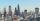The distance between two cities is actually 30 km and the map is 6 cm. What is the scale of the map?
4. Milk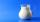At the kindergarten, every child got 1/5 liter of milk in the morning and another 1/8 liter of milk in the afternoon. How many liters were consumed per day for 20 children?
5. Cube cutIn the ABCDA'B'C'D'cube, it is guided by the edge of the CC' a plane witch dividing the cube into two perpendicular four-sided and triangular prisms, whose volumes are 3:2. Determine in which ratio the edge AB is divided by this plane.
6. Boys to girlsThe ratio of boys to girls in a party is 3:5 . If 6 more boys arrived and 4 girls left the party, the ratio of boys to girls would be 5:6 . How many are in the party originally?
7. Red diplomasHe numbers of students with honors in 2013 and 2014 are in ratio 40:49. How big is the year-on-year percentage increase?
8. The sidesThe sides of a rectangle are in a ratio of 2:3, and its perimeter is 1 1/4 inches. What are the lengths of its side? Draw it.
9. Mixed2improperWrite the mixed number as an improper fraction. 166 2/3
10. Sum of fractionsWhat is the sum of 2/3+3/5?
11. Sewing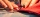Beth's mother can sew 235 pairs of short pants in 6 days while Lourdes can sew 187 pairs in 8 days. How many more pairs of short pants can Beth's mother sew?
12. Sewing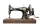The lady cut off one half of cloth. She needed three-quarters of this piece to sew a skirt. What part of the original piece of cloth still remained?
13. New ratio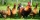The ratio of ducks and chicken in our yard is 2 : 3. The total number of ducks and chickens together is 30. Mother gave 3 of the chickens to our neighbor. What is the new ratio now?
14. Wiring 2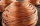Willie cut a piece of wire that was 3/8 of the total length of the wire. He cut another piece that was 9m long. The two pieces together were one half of the total length of the wire. How long was the wire before he cut it?
15. SSA and geometryThe distance between the points P and Q was 356 m measured in the terrain. The PQ line can be seen from the viewer at a viewing angle of 107° 22 '. The observer's distance from P is 271 m. Determine the viewing angle of P and observer.
16. Equation - inverseSolve for x: 7: x = 14: 1000
17. Soup from canteen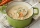For how many people is 90 liters of soup enough if we assume 3/8 liter of soup per person in the canteen?
18. Marriage sttusIn our city, there are 3/5 of the women married to 2/3 of the men. Find what part of the population is free.
19. Simple equation 5Solve equation with fractions: X × 3/8 = 1/2
20. Three segments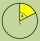The circle is divided into 3 segments. Segment A occupies 1/4 of the area, segment B occupies 1/3 of the area. What part is occupied by section C? In what proportion are areas A: B: C?

Do you have an interesting mathematical word problem that you can't solve it? Enter it, and we can try to solve it.

To this e-mail address, we will reply solution; solved examples are also published here. Please enter e-mail correctly and check whether you don't have a full mailbox.

Need help calculate sum, simplify or multiply fractions? Try our fraction calculator.